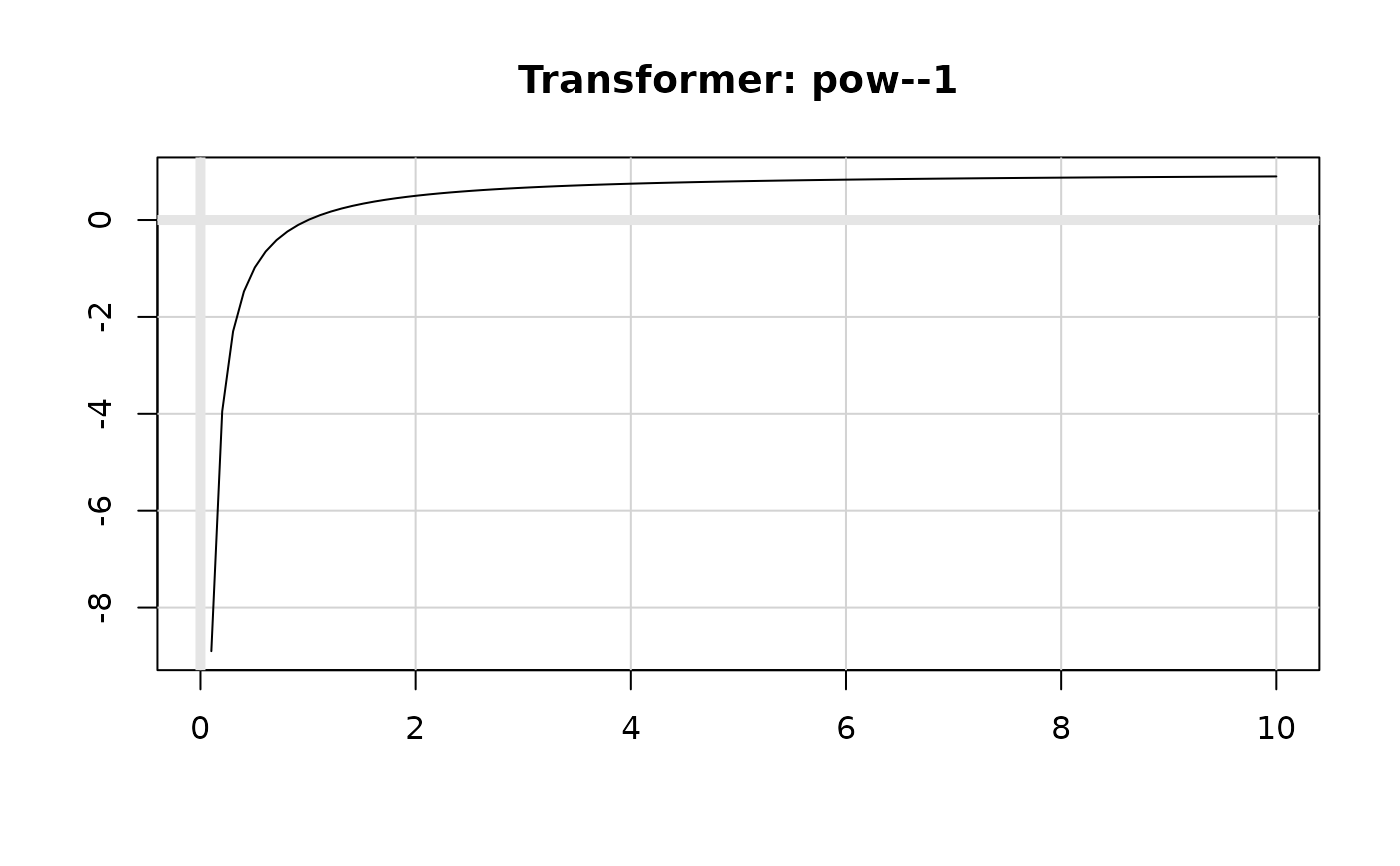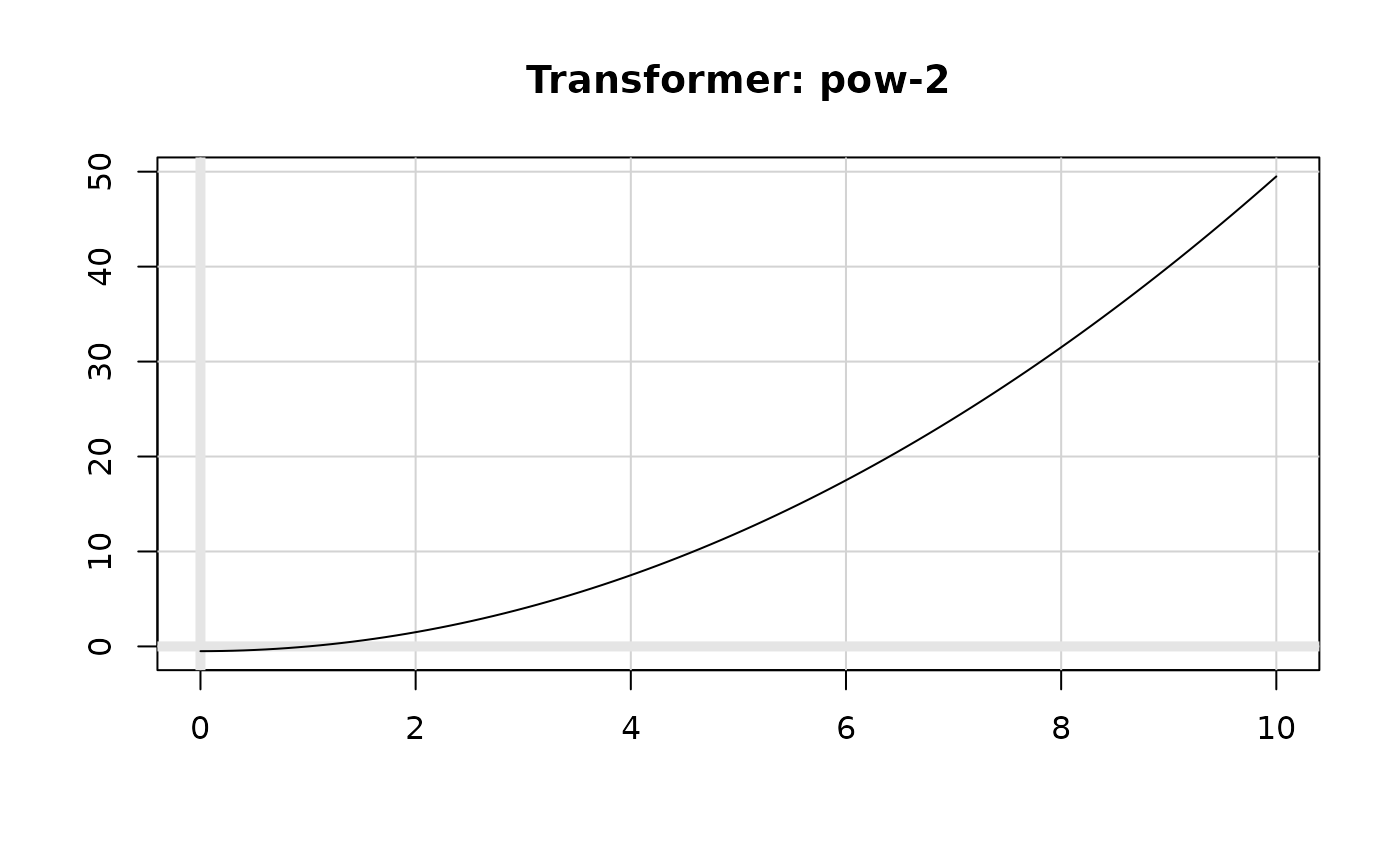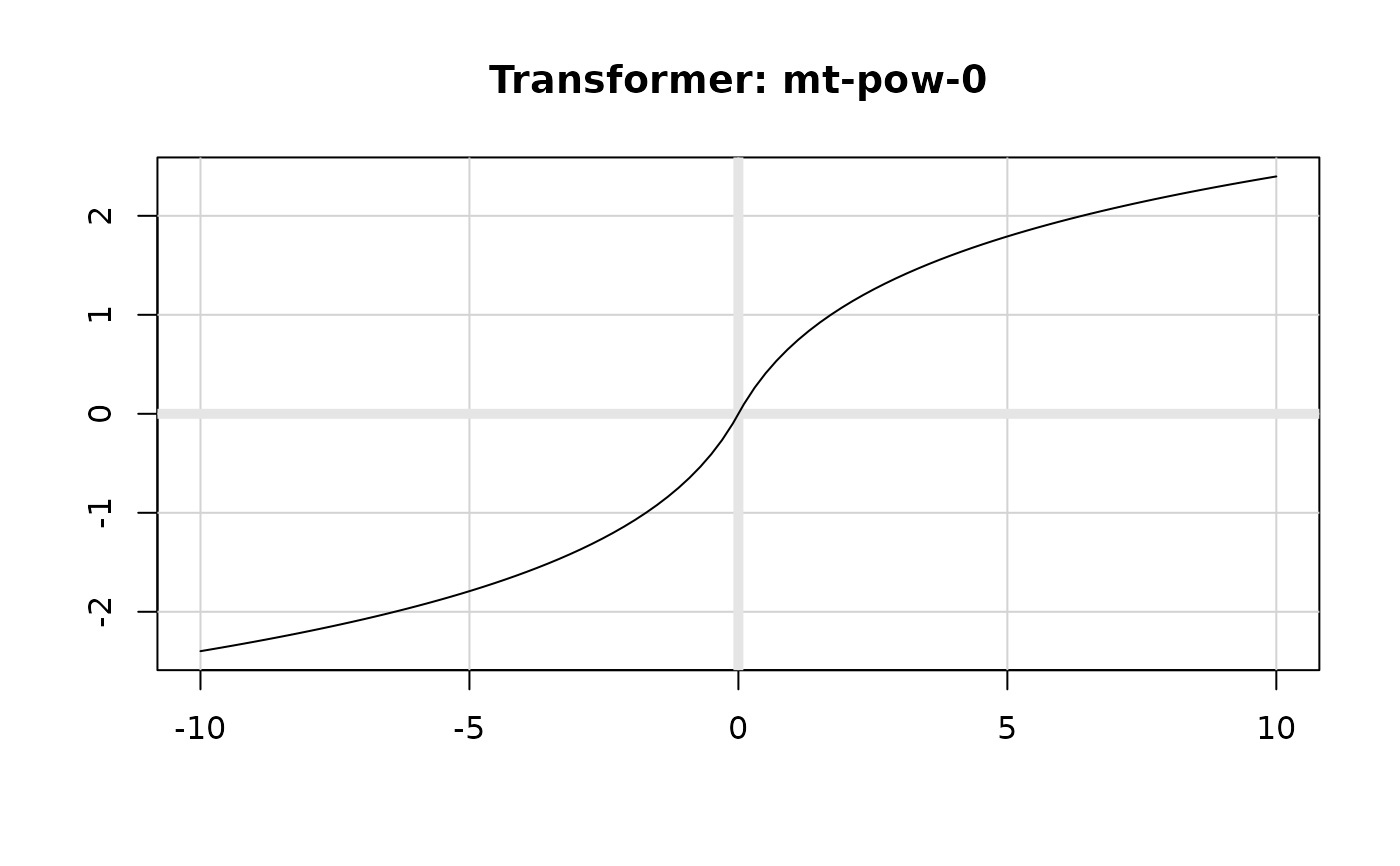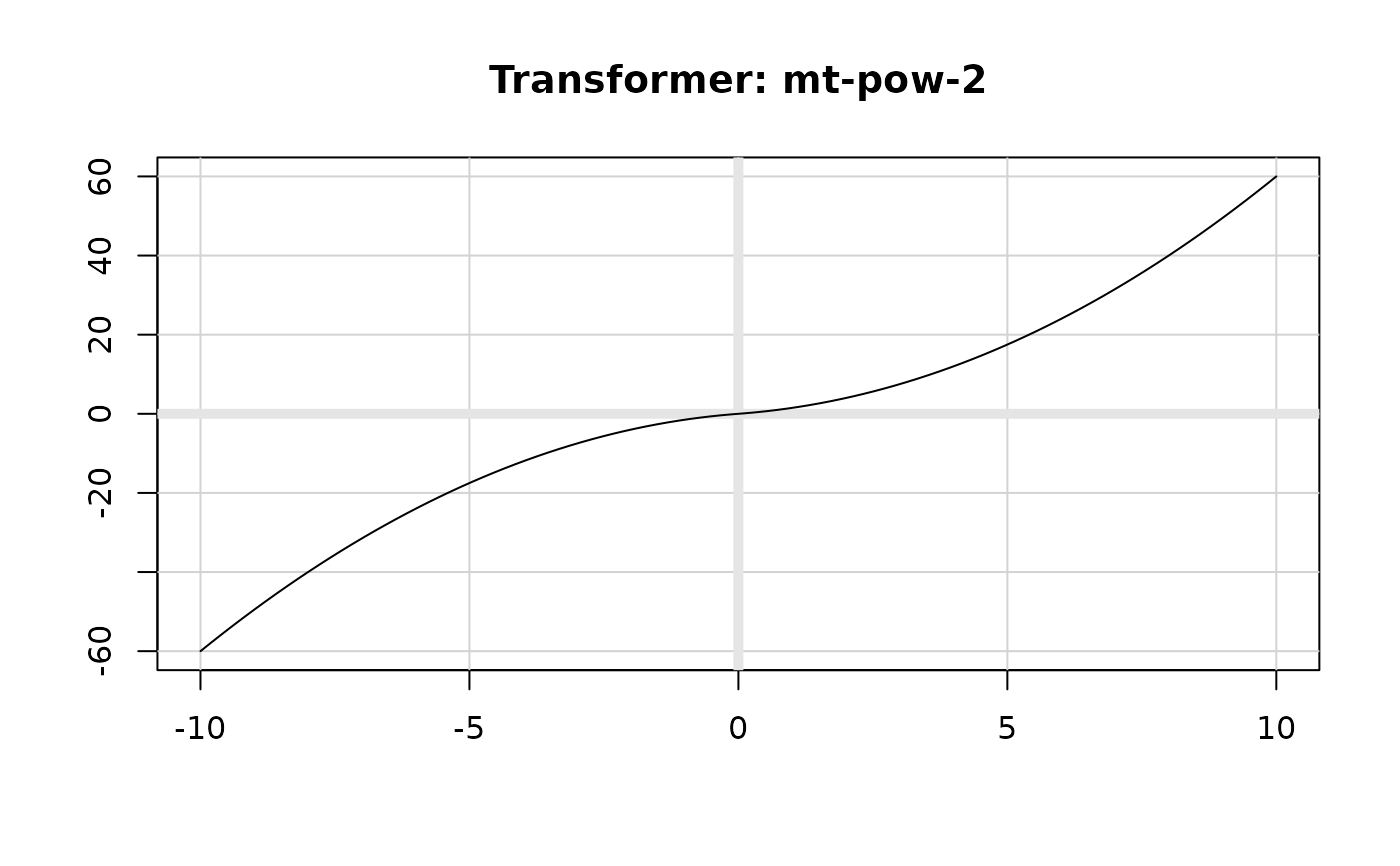The Box-Cox transformation is a flexible transformation, often used to transform data towards normality. The modulus transformation generalises Box-Cox to also work with negative values.

boxcox_trans(p, offset = 0)

modulus_trans(p, offset = 1)

## Arguments

p Transformation exponent, $$\lambda$$. Constant offset. 0 for Box-Cox type 1, otherwise any non-negative constant (Box-Cox type 2). modulus_trans() sets the default to 1.

## Details

The Box-Cox power transformation (type 1) requires strictly positive values and takes the following form for y > 0: $$y^{(\lambda)} = \frac{y^\lambda - 1}{\lambda}$$ When y = 0, the natural log transform is used.

The modulus transformation implements a generalisation of the Box-Cox transformation that works for data with both positive and negative values. The equation takes the following forms, when y != 0 : $$y^{(\lambda)} = sign(y) * \frac{(|y| + 1)^\lambda - 1}{\lambda}$$ and when y = 0: $$y^{(\lambda)} = sign(y) * \ln(|y| + 1)$$

Box, G. E., & Cox, D. R. (1964). An analysis of transformations. Journal of the Royal Statistical Society. Series B (Methodological), 211-252. https://www.jstor.org/stable/2984418

John, J. A., & Draper, N. R. (1980). An alternative family of transformations. Applied Statistics, 190-197. http://www.jstor.org/stable/2986305

## See also

yj_trans()

## Examples

plot(boxcox_trans(-1), xlim = c(0, 10))plot(boxcox_trans(0), xlim = c(0, 10))plot(boxcox_trans(1), xlim = c(0, 10))plot(boxcox_trans(2), xlim = c(0, 10))plot(modulus_trans(-1), xlim = c(-10, 10))plot(modulus_trans(0), xlim = c(-10, 10))plot(modulus_trans(1), xlim = c(-10, 10))plot(modulus_trans(2), xlim = c(-10, 10))